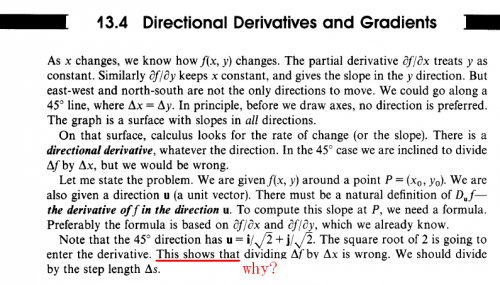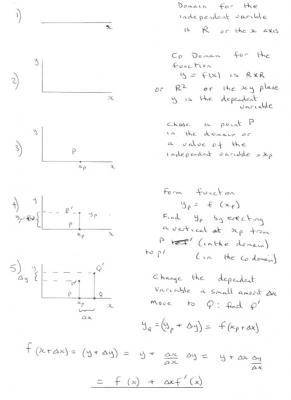## Recommended Posts

I am learing directional derivative and one context in the book puzzle me, i know delta f should be divided by

delta s not delta x when we use directional derivative,but i really can't understand the explain in the book.

Anyone can help me ?Edited by bingliantech

##### Share on other sites

Small s is the usual symbol for arc length and refers to length measured along the curve.

So delta s is a small change in distance (and therefore position along the curve.

delta x and delta y can be thought of as the components of delta s.

So point s1 is at (x1y1) and s2 is at (x2y2)

The line from s1 to s2 is a short section of the curve ie along the curve.

that is why we call this the directional derivative.

Sorry I don't have time for a diagram at the moment, but will do later this morning.

Does this help.

##### Share on other sites

Thank you for your reply, i can understand what you have said as i can understand the first three paragraghs in the picture,but the last paragraph puzzle me,i can't get why the square root of 2 going to enter shows that dividing delta f by delta x is wrong.

##### Share on other sites

I am rather at a loss to understand why anyone would want to divide

$\Delta f\;by\;\Delta x$

as stated in paragraph 2.

The text is clearly referring to the 3D situation where z = f(x,y).

that is values of the function f(x,y) are plotted as a surface as values on the z axis.

It starts off in paragraph 1 by saying just that and that the result is a surface.

In fact it hints at the truth which is that even the partial derivatives
$\frac{{\partial f}}{{\partial x}}\;and\;\frac{{\partial f}}{{\partial y}}$
are directional derivatives, the first in the x direction and the second in the y direction.

Clearly any other direction has components from both x and y.

The point P(x0,y0) is in the plane z=0, as are the steps
$\Delta x$, $\Delta y$ and$\Delta s$

In fact you can take a short $\Delta s$ step in any direction, but you are still in the plane z=0 or the domain of x-y.

The chain rule tells us that

$\frac{{df}}{{ds}} = \frac{{\partial f}}{{\partial x}}\;\frac{{dx}}{{ds}} + \frac{{\partial f}}{{\partial y}}\;\frac{{dy}}{{ds}}$

##### Share on other sites

Agree with Studiot . I think the book is hinting at the conceptual basis for the derivative the limit as the step tends to zero, but wants to make sure that you are taking the limit of the correct ratio ie stepping in the right direction. Any step delta x will be root 2 bigger than the correct step delta s - I presume he is using big delta as there is a tendency for all dx to be seen as the same in the limit.

$\frac{{\partial f}}{{\partial x}}$ is the directional derivative along the unit vector <1,0> which if you look at Studiots final chain rule equation makes sense.

I always find Paul's Online Notes brilliant for Calculus problems

http://tutorial.math.lamar.edu/Classes/CalcIII/DirectionalDeriv.aspx

edit ignore the <spans>

##### Share on other sites

Thank you very much, just as you said, the authour may want to say if we divide f by x , we will get root 2* f/s for limit,only f/s is right, i am not very customed with the expression in the last paragragh.

Thanks a lot for studiot and imatfaal's help and these paragraphs come from strang's "calculus".

##### Share on other sites

I have not looked a Strang's Calculus before but have heard a few adverse comments.

I did find it it patchy with some quite deep material interspersed with some very superficial treatment, despite the 671 pages.

His coverage is much wider than attempted by most authors but many of the little intermediate steps and explanations are missing, so I feel would be any course using this book.

Unfortunately this applies to section 13 which you are referring to.

In particular he has mixed the vector based approach with the traditional geometric one, omitting important parts of both.

We can discuss this further if you wish.

Are you familiar with the vector dot product?

##### Share on other sites

Well his Linear Algebra is - in my opinion - brilliant. He is very odd though and I seem to remember having some misgivings about his linear algebra until I decided to forget my current knowledge and trust that following his ideas would work - and after a bit of head-scratching and a huge amount of his practice questions I realised that it was my initial understanding which was flawed and I had done well to go back to a more basic level.

I think both his introduction to Linear Algebra and Intro to Calculus is available on MIT Openware. That is to say videos of the full lecture course that those books were written to accompany.

If you are struggling with any concept in the book - the lecture of the man that wrote it will always help.

Sorry
- just rechecked

http://ocw.mit.edu/courses/find-by-topic/#cat=mathematics&subcat=calculus

Gilbert's course is not up there - but others are.

The book is here

http://ocw.mit.edu/resources/res-18-001-calculus-online-textbook-spring-2005/

The chapter on partials is here

http://ocw.mit.edu/resources/res-18-001-calculus-online-textbook-spring-2005/textbook/MITRES_18_001_strang131134.pdf

The linear Algebra course is up there as videos

##### Share on other sites

In particular he has mixed the vector based approach with the traditional geometric one, omitting important parts of both.

We can discuss this further if you wish.

Are you familiar with the vector dot product?

Yes,i found Strang's linear algebra is very good and it's the reason why i want reading his calculus carefully.

I generally read his linear algebra several days ago so i think i may have the basic understanding of vector dot product.

Could you tell me the omiting parts ?I can understand f should be devided by s,it's not difiicult to be understood,i just be puzzled with his reason or his explanation.

to imatfaal

It's a good idea to watch the video of lecture,but i don't listen english often,maybe i can make a try.

Thank you!

##### Share on other sites

OK so here is the first part of what I think Strang means.

I will try to keep to short sentences.

It is very important to understand what variable are and what a function is.

So I have started with the simplest.

A variable can be dependent or independent.

The simplest function has one of each type.

A function also has a domain and a co-domain.

They are not always the same.

The domain is the set that includes all the values of the independent variable(s), the co-domain includes all the values of the dependent variable.

I am going to use R, the set of all Real numbers as the domain (independent variable).

In other words the x axis.

This is fig(1) in my sketch.

In order to draw a graph and construct a function y = f(x) I need to have a co-domain that can hold number pairs (x, y).

This is called R2 or RxR and ie represented by the xy plane.

y is the dependent variable.

This is fig(2) in my sketch.

To construct our function we choose a point P in the domain with value, xp

This is fig(3) in my sketch.

We calculate the value of y at x=xp as yp =f(xp) and plot P' in the co-domain.

This is fig(4) in my sketch.

If we now move to another value Q of the independent variable, x, with a small change $\Delta x$, we can calculate the change in the dependent variable resulting from the change in the independent variable.

Now in this simple case there is only one direction of change for the independent variable (ignoring sign).

That is along the x axis.

So the f'(x) that we calculate by the formulae in my fig (5) is the directional derivative for this case.

It depends only on $\Delta x$

This is what Strang meant by we might expect to divided by $\Delta x$

When we look at the case of two independent variables in the next instalment, things are more complicated.

The domain is now R2 not R and the co-domain now R3 not R2. (can you see a pattern here?)

But we must go through the same steps 1 - 5.

Please confirm that you have understood this so that we can proceed.##### Share on other sites

OK,no problem,i considered M which is {y1,y2,y3..yp...}⊂M as co-domain in the past,i can understand your steps and i think i can understand partial derivative.

## Create an account

Register a new account